Test: Capacitors & Inductors

# Test: Capacitors & Inductors

Test Description

## 10 Questions MCQ Test GATE Electrical Engineering (EE) 2023 Mock Test Series | Test: Capacitors & Inductors

Test: Capacitors & Inductors for Electrical Engineering (EE) 2022 is part of GATE Electrical Engineering (EE) 2023 Mock Test Series preparation. The Test: Capacitors & Inductors questions and answers have been prepared according to the Electrical Engineering (EE) exam syllabus.The Test: Capacitors & Inductors MCQs are made for Electrical Engineering (EE) 2022 Exam. Find important definitions, questions, notes, meanings, examples, exercises, MCQs and online tests for Test: Capacitors & Inductors below.
Solutions of Test: Capacitors & Inductors questions in English are available as part of our GATE Electrical Engineering (EE) 2023 Mock Test Series for Electrical Engineering (EE) & Test: Capacitors & Inductors solutions in Hindi for GATE Electrical Engineering (EE) 2023 Mock Test Series course. Download more important topics, notes, lectures and mock test series for Electrical Engineering (EE) Exam by signing up for free. Attempt Test: Capacitors & Inductors | 10 questions in 30 minutes | Mock test for Electrical Engineering (EE) preparation | Free important questions MCQ to study GATE Electrical Engineering (EE) 2023 Mock Test Series for Electrical Engineering (EE) Exam | Download free PDF with solutions
 1 Crore+ students have signed up on EduRev. Have you?
Test: Capacitors & Inductors - Question 1

### An Inductor works as a ________ circuit for DC supply.

Detailed Solution for Test: Capacitors & Inductors - Question 1

Induced voltage across an inductor is zero if the current flowing through it is constant, i.e. Inductor works as a short circuit for DC supply.

Test: Capacitors & Inductors - Question 2

### The equivalent circuit of the capacitor shown below is: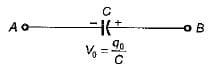Detailed Solution for Test: Capacitors & Inductors - Question 2

Due to initial condition, at t = 0 capacitor will act as a constant voltage source (at t = 0, capacitor acts as short-circuit). Hence, option (d) is correct.

Test: Capacitors & Inductors - Question 3

### The strength of current in 1 Henry inductor changes at a rate of 1 A/sec. The magnitude of energy stored in the inductor after 3 sec is:

Detailed Solution for Test: Capacitors & Inductors - Question 3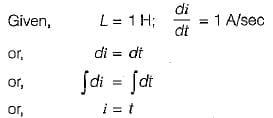∴ Current in the inductor after 3 sec is:

1= 3 A

Hence, energy is stored after 3 sec: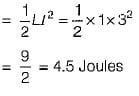Test: Capacitors & Inductors - Question 4

The current and voltage profile of an element vs time has been shown in given figure. The element and its value are respectively: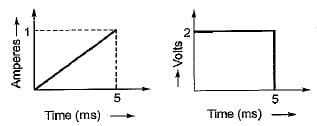Detailed Solution for Test: Capacitors & Inductors - Question 4
• Since V is not proportional to R therefore, the element can’t be a resistor.
• At t = 5 ms, even if i ≠ 0, the element behaves as a short circuit therefore, the element can’t be a capacitor (since at t = 0 only capacitor behaves as short circuit).
• The current at t = 0 is zero and at t = 5 ms voltage across the element is zero therefore, the element must be an inductor (at t = 0, an inductor acts as open circuit and at t =∞ it behaves as short circuit).
• From the given voltage and current profile, we have: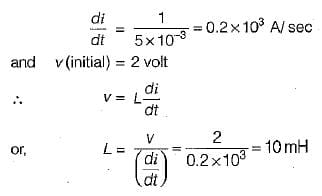Test: Capacitors & Inductors - Question 5

Figure shown below exhibits the voltage-time profile of a source to charge a capacitor of 50 μF. The value of charging current in amperes is: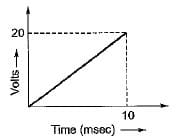Detailed Solution for Test: Capacitors & Inductors - Question 5

From given figure: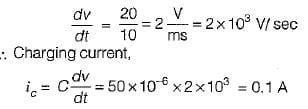Test: Capacitors & Inductors - Question 6

The equivalent capacitance across the given terminals A-B is: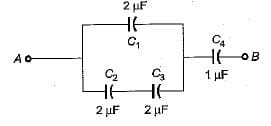Detailed Solution for Test: Capacitors & Inductors - Question 6
• The equivalent combination of C2 and C3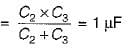• The equivalent combination of this 1 μF and  C1 = 2μF is C1+ 1 μF = 3μF
• Hence, the equivalent capacitance between terminals A and B is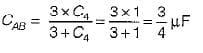Test: Capacitors & Inductors - Question 7

The charging time required to charge the equivalent capacitance between the given terminals a-b by a steady direct current of constant magnitude of 10 A is given by: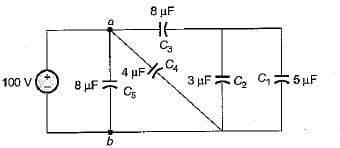Detailed Solution for Test: Capacitors & Inductors - Question 7

Equivalent capacitance between terminals a-b is: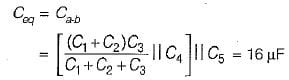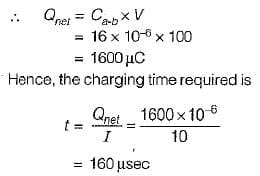Test: Capacitors & Inductors - Question 8

An ac voltage of 220 V is applied to a pure inductance of 50 H. If the current is 5 A, the instantaneous value of voltage and current will be respectively given by:

Detailed Solution for Test: Capacitors & Inductors - Question 8

Max. value of current: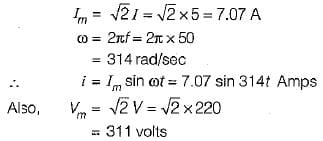Assuming voltage as the reference phasor: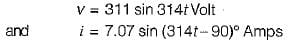Test: Capacitors & Inductors - Question 9

The voltage and current through a circuit element is v= 100 sin (314 t + 45°) volts and i = 10 sin (314 t - 45 ° ) amps.
The type of circuit element and its value will be respectively:

Detailed Solution for Test: Capacitors & Inductors - Question 9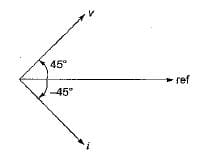The phase difference between v and i is: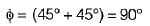Since v leads i therefore, the circuit element is an inductor.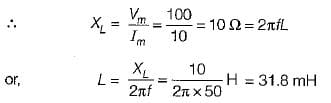Test: Capacitors & Inductors - Question 10

The equivalent inductance for the inductive circuit shown below at terminal “ 1 - 2 ” is: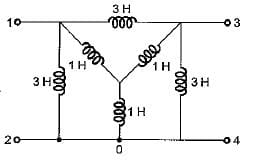Detailed Solution for Test: Capacitors & Inductors - Question 10

Converting the internal star connected inductance to an equivalent delta, the circuit reduces as shown below.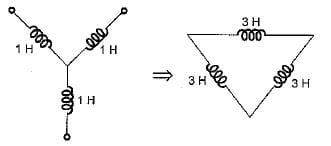Hence, equivalent circuit becomes as shown below.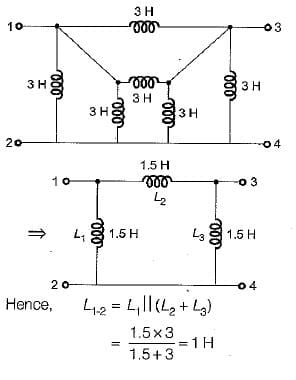## GATE Electrical Engineering (EE) 2023 Mock Test Series

18 docs|208 tests
 Use Code STAYHOME200 and get INR 200 additional OFF Use Coupon Code
Information about Test: Capacitors & Inductors Page
In this test you can find the Exam questions for Test: Capacitors & Inductors solved & explained in the simplest way possible. Besides giving Questions and answers for Test: Capacitors & Inductors, EduRev gives you an ample number of Online tests for practice

## GATE Electrical Engineering (EE) 2023 Mock Test Series

18 docs|208 tests